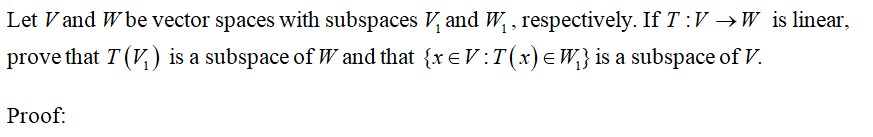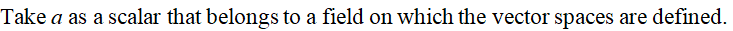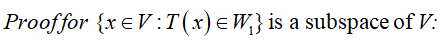# Let V and W be vector spaces with subspaces V1 and W1, respectively. If T: V → W is linear, prove that T(V1) is a subspace of W and that {x ∈ V: T(x) ∈ W1} is a subspace of V.

Question
75 views

Let V and W be vector spaces with subspaces V1 and W1, respectively. If T: V → W is linear, prove that T(V1) is a subspace of W and that {x ∈ V: T(x) ∈ W1} is a subspace of V.

check_circle

Step 1Step 2Step 3...

### Want to see the full answer?

See Solution

#### Want to see this answer and more?

Solutions are written by subject experts who are available 24/7. Questions are typically answered within 1 hour.*

See Solution
*Response times may vary by subject and question.
Tagged in